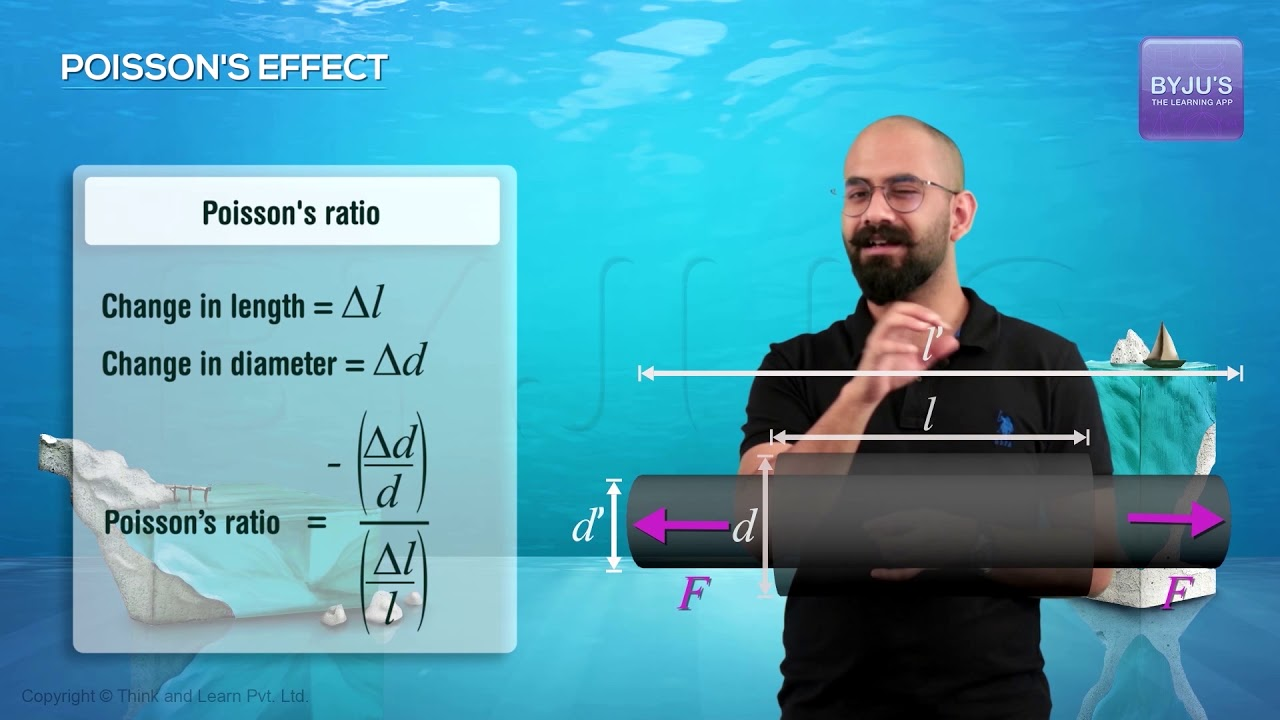Checkout JEE MAINS 2022 Question Paper Analysis : Checkout JEE MAINS 2022 Question Paper Analysis :

# Bulk Modulus Formula Derivation

## Bulk Modulus

Bulk modulus of a substance is defined as the ratio of infinitesimal pressure increase to a decrease of the volume. Bulk modulus is meaningful only for a fluid. It is denoted as either K or B. The reciprocal of bulk modulus is compressibility of a substance and this is the relation between bulk modulus and compressibility.

The mathematical representation of bulk modulus is given as follows:

For bulk modulus K > 0;

$$\begin{array}{l}K=-V\frac{dP}{dV}\end{array}$$

Where,

P: pressure

V: volume

$$\begin{array}{l}\frac{dP}{dV}:derivative\, of \, pressure \, with \, respect \, to \, volume\end{array}$$

### Bulk Modulus For Unit Mass

$$\begin{array}{l}K=\rho \frac{dP}{d\rho }\end{array}$$

Where,

⍴: density

$$\begin{array}{l}\frac{dP}{dV}: derivative \, of \, pressure \, with \, respect \, to \, density\end{array}$$
Bulk modulus in thermodynamics with constant temperature and constant entropy is given as follows:

Isentropic bulk modulus Ks;

$$\begin{array}{l}K_{s}=\gamma p\end{array}$$

Isothermal bulk modulus KT;

$$\begin{array}{l}K_{T}= p\end{array}$$

Where,

p: pressure

γ: heat capacity ratio

Following is the table of bulk modulus of a few common materials:

 Material Bulk modulus in GPa Diamond 443 Rubber 1.5 to 2 Steel 160

## Bulk Modulus Formula Derivation

As we know the ratio between change in pressure to change in volumetric strain is dependent on bulk modulus of the material, following is the derivation showing the relationship:

$$\begin{array}{l}\frac{-\delta V}{V}=\frac{\delta p}{K}\end{array}$$
(negative sign to indicate that with increase in pressure, volume decreases)

Where,

δV: change in volume

δp: change in pressure

V: actual volume

K: bulk modulus

$$\begin{array}{l}K=-V\frac{dp}{dV}\end{array}$$
(as δp tends to 0) (eq.1)

$$\begin{array}{l}V=\frac{1}{\rho }\end{array}$$
(unit mass of the substance) (eq.2)

$$\begin{array}{l}Vd\rho +\rho dV=0\end{array}$$
(after differentiation)

$$\begin{array}{l}dV=-(\frac{V}{\rho })d\rho\end{array}$$
(eq.3)

$$\begin{array}{l}K=\frac{-Vdp}{-(\frac{V}{\rho })d\rho }\end{array}$$
(after substituting eq.3 in eq.1)

$$\begin{array}{l}∴ K=\rho \frac{dP}{d\rho }\end{array}$$

Thus, above is the derivation of bulk modulus which is mainly applicable to liquids as gases are highly compressible which makes K vary.

## Solved Example

Q1. What is the bulk modulus of a body that experienced a change of pressure of 5 x 104 N/m2 and its volume goes from 4 cm3 to 3.9 cm3?

Solution: The bulk modulus is calculated using the formula,
B = ΔP /(ΔV/V)
B = (5 x 104 N/m2)/((4 cm3 – 3.9 cm3)/4 cm3) = 0.125 x 104 N/m2
B = 1.25 x 104 N/m2

Q2. In the ammunition testing centre the pressure is found to be 255 MPa. Calculate the change in volume of the copper piece when subjected to this pressure in percentage. The bulk modulus of copper is 1.38 x 1011 Pa.

Solution
The pressure in the testing centre is 255 MPa.
Bulk modulus, K = V(∆P) / ∆V
Substituting the values,
(ΔV / V) = 255 × 106/ 1.38×1011 × 100
Therefore, the change in volume percentage is 0.184 %

To know more on other Physics related concepts, stay tuned with BYJU’S.

Related Physics articles:

## Watch the video and understand Poisson’s Ratio.## Frequently Asked Questions – FAQs

### What is meant by bulk modulus?

The bulk modulus of a body is the ratio of infinitesimal pressure increase to a decrease of the volume.

### What is known as the reciprocal of bulk modulus?

The compressibility of a body is the reciprocal of the bulk modulus.

### What is the bulk modulus of a diamond?

443 GPa is the bulk modulus of a diamond.

### What is the bulk modulus of rubber?

1.5 to 2 GPa is the bulk modulus of rubber.

### What are the main quantities that control bulk modulus?

Pressure and volume.
Test your knowledge on Bulk modulus formula derivation